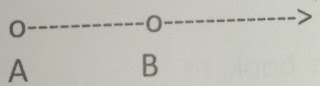# Lesson Planning of MEASUREMENT OF ANGLE

Lesson Planning of MEASUREMENT OF ANGLE WITH PROTRACTOR

Subject Mathematics

Students` Learning Outcomes

• Draw a right angle using protractor.
• Draw acute and obtuse angles of different measures using protractor.
• Draw an angle using protractor:
1. Equal in measure to a given angle,
2. Twice the measure of a given angle,
3. Equal in measure of the sum of two given angles,

Information for Teachers

• To draw an angle of 50° first draw a line segment angle will be draw at its one end.• Then place the protractor so that its zero line matches with your line segment and that the vertex is in correct place. Then put a little mark at the spot showing 50 degrees.
• Take the protractor off and draw a line through your mark till vertex point.
• During this lesson teacher should consult textbook wherein and whenever it is applicable.

Material / Resources

Writing board, chalk/marker, duster, geometry box, pencil, rubber, paper, textbook

Introduction

• Recall the drawing of vertical line by using set-squares.
• Ask any of the students to repeat and then introduce another way of drawing it.

Development

 Activity 1 Say: “We can also use a protractor to construct a right angle.” Using the straight edge of the protractor, draw rayon board, as;Say: “We are going to construct a right angle with its vertex at point “A”. How should we place the central point of the protractor?” (Place the central point of the protractor on point “A” and the 0 line of the protractor on ray AB.) Ask a student to demonstrate this at the board with the large wooden protractor.Ask the students, which mark on the scale will represent right angle? (90°) Ask a student to place a point on the board at the 90° mark, label it point C. remove the protector and draw a ray from point “A” through point “C”. Ask: “How can we be ascertaining that we have constructed right angle? (It measures 90° we can match against a known right angle, such as the corner of a page in a book). What are the correct names for this angle?” (We can call it angle LA, angle ∠BAC, or angle ∠CAB). Ask a student to write the angle names on the board as the others write them in their notebooks. Repeat the procedure by constructing additional angles. Assign practice questions from book to work in individually.

Sum up / Conclusion
• Right angle is of 90°.
• Acute angle is greater than 0° and less than 90°.
• Obtuse angle is between 90° and 180°.
• Protractor is used to draw all angles.

Half or twice of an angle can be draw with protractor.

Assessment

• Ask them to draw 5 angles of their own choice and label them.
• Any student come on board and demonstrates drawing of right angle.